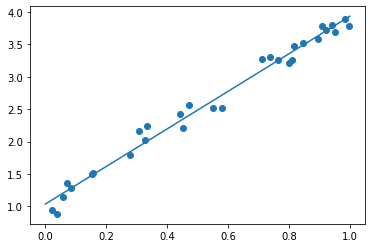# MOSEK in Python online demo¶

You can run this notebook in Google Colab (or any other environment that provides !pip install) as is. There is no need to install a license or anything else.

The optimization will be performed on https://solve.mosek.com/.

In [ ]:
# Install MOSEK if not already installed
!pip install mosek

In [ ]:
# In this example we use the Fusion API
#    https://docs.mosek.com/latest/pythonfusion/index.html
# One could also use the lower level Optimizer API for Python
#    https://docs.mosek.com/latest/pythonapi/index.html
from mosek.fusion import *
import sys
import numpy as np

# Set up a very simple linear regression problem for demonstration
def mosek_least_squares(m, n, A, b, log):
M = Model()
x = M.variable(n)
t = M.variable()

# t >= ||Ax-b||_2
M.constraint(Expr.vstack(t, Expr.sub(Expr.mul(A, x), b)),
Domain.inQCone())

M.objective(ObjectiveSense.Minimize, t)

# Use the online demo optimization server
M.optserverHost("http://solve.mosek.com:30080")
M.setLogHandler(sys.stdout if log else None)
M.solve()

print("Problem status: {prosta}".format(prosta=M.getProblemStatus()))
print("residual: {t}".format(t=t.level()))

if M.getProblemStatus() == ProblemStatus.PrimalAndDualFeasible:
return x.level()

In [ ]:
# Random example
m, n = 20, 15
A = np.random.randn(m, n)
b = np.random.randn(m)

x = mosek_least_squares(m, n, A, b, False)

Problem status: ProblemStatus.PrimalAndDualFeasible
residual: 1.5633248125910382

In [ ]:
# 2-dimensional example with intercept
m = 30
X = np.random.rand(m)
Y = 3 * X + 1 + 0.5*(np.random.rand(m)-0.5)

a = mosek_least_squares(m, 2, np.vstack((X,np.ones(m))).transpose(), Y, False)

print("Regression line: {0:.3f} x + {1:.3f}".format(a, a))
%matplotlib inline
import matplotlib.pyplot as plt
d = np.linspace(0, 1, 2)
plt.scatter(X, Y)
plt.plot(d, a * d + a)

Problem status: ProblemStatus.PrimalAndDualFeasible
residual: 0.689564246467142
Regression line: 2.902 x + 1.034

Out[ ]:
[<matplotlib.lines.Line2D at 0x7f87327f9ac8>]# What now?¶

The demo service at https://solve.mosek.com has size limitations. If you want to use MOSEK on your own grab and install a trial or academic license and remove the call to M.optserverHost to optimize locally.

Alternatively, install your own OptServer, install a license there, and redirect to it in the M.optserverHost call.